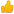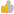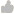> Knowledge base> Article: How is cost per label calculated in the CX1200 Status Monitor?

# How is cost per label calculated in the CX1200 Status Monitor?

Article ID: 44923Question
How is cost per label calculated in the CX1200 Status Monitor?

How is cost per label calculated in the CX1200 Status Monitor?

1.

Cost is determined starting with 4 fixed yield values from each of the cartridges. The yield is the number of printed pages you will receive out of each cartridge at 5% coverage per page. These values were derived from testing hundreds of cartridges using various 5% coverage images. 5% coverage is NOT a typical print coverage. However, as you will see, for the purposes of toner cost calculation this does not matter.

Total Cartridge Yield

Cyan -- 16500

Magenta -- 16500

Yellow -- 16500

Black -- 16500

2.

The next step is to find the actual percent coverage value reported by the printer for any particular print. To get these values we use the Percent Used column of the CX1200 Status Monitor quote tool tab. The percent used values are determined by printer engine which analyzes each page using internal software.

3.

Next we calculate the deviation from the 5% coverage value that is used to determine the total yield of each color. To do this we divide the percentage reported in the Percent Used column by 5. To get this number we use whole numbers instead of percents.

Actual Coverage / 5 = Coverage Multiplier

Cyan = 10%

10 / 5 = 2

Magenta = 8%

8 / 5 = 1.6

Yellow = 3%

3 / 5 = .6

Black = 6%

6 / 5 = 1.2

These values would represent the toner used that is above or below the 5% that printer engine reports.

4.

At this point we would calculate the updated number of pages that a cartridge would yield for this label.

Total Cartridge Yield / Coverage Multiplier = Updated Expected Pages for this label

Cyan

16500 / 2 = 9900 pages

Magenta

16500 / 1.6 = 10312 pages

Yellow

16500 / .6 = 27500 pages

Black

16500 / 1.2 = 13750 pages

5.

Now we determine the cost per label per cartridge.

Cost of Cartridge / Updated Expected Pages = Cost per label per Cartridge

Cyan

425 / 9900 = $.042\label Magenta 425 / 10312 =$.041\label

Yellow

425 / 27500 = $.015\label Black 189 / 13750 =$.013\label

6.

Finally add all costs per label per cartridge together to get total cost per label.

Cyan Cost per label + Magenta Cost per label + Yellow Cost per label + Black Cost per label = Total Label Cost

Total Label cost would be \$0.111 (11 cents).

7. Since this job only had a single label per page we would not need to further divide the cost of each color. Had there been multiple labels per page you would divide the Total Page Cost by the number of labels on that page.

Total Page Cost / Number of labels on that page = Total Label Cost when printing multiple labels per page

related articles

Article Details
 Views: 3317 Created on: May 24, 2014 Date updated: Apr 27, 2016 Posted in: CX1200-FX1200-CX1000-FX1000

 Poor 12345 Outstanding